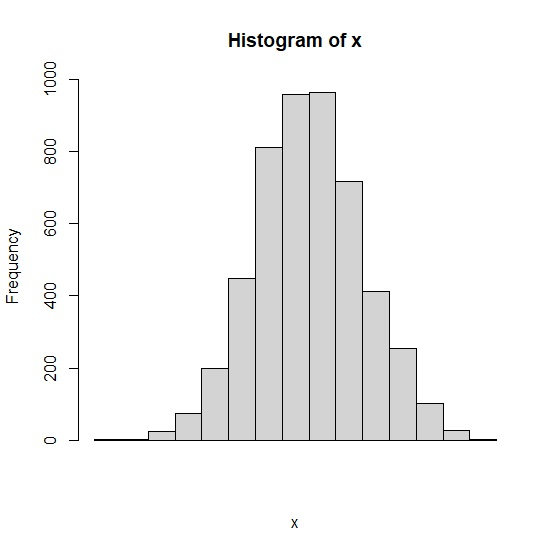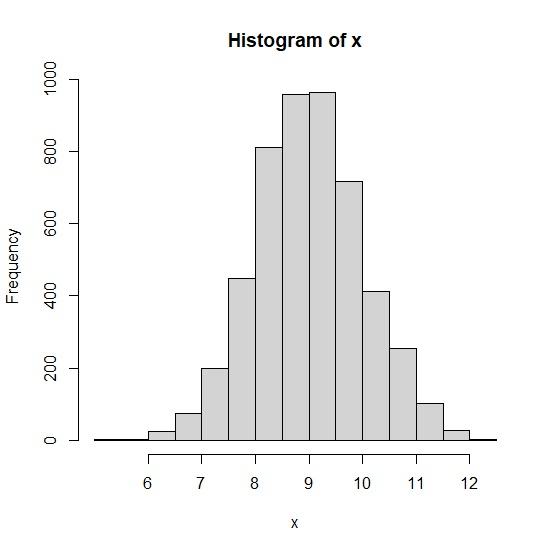# How to apply manually created x-axis labels in a histogram created by hist function in R?

When we generate a histogram in R using hist function, the x-axis labels are automatically generated but we might want to change them to values defined by researchers or by any other authority. Therefore, firstly we need to create the histogram by ignoring the labels and then axis function can be used for new values.

Consider the below vector x and create a histogram of x by ignoring x-axis labels −

## Example

set.seed(1999)
x<-rnorm(5000,9,1)
hist(x,xaxt='n')

## OutputNow adding new values for x-axis labels −

## Example

axis(side=1,at=seq(6,12,1),labels=seq(6,12,1))

## Output